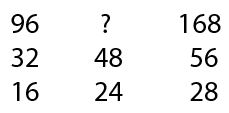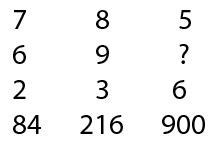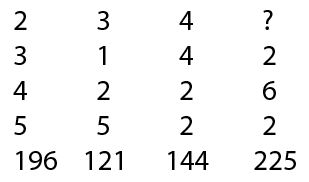# SSC CGL 2014 Tier 1 19 Oct shift 1

Instructions

For the following questions answer them individually

Question 1

Which of the following interchange of signs would make the equation correct ?
8 × 6 +2 =22

Question 2

If ‘-‘ stands for addition, ‘+’ stands for subtraction, ‘÷’ stands for multiplication and ‘×’ stands for division, then which one of the following equation is correct ?

Instructions

In each of the following questions, some equations are solved on the basis of certain system. Find out the correct answer for the unsolved equation on that basis.

Question 3

2 × 4 × 6 = 4; 9 x 3 x 7 = 13;
4 × 7 × 6=3; 9 × 7 × 8 =?

Question 4

3 × 5 × 7 × 2 = 24, 2 × 4 × 6 × 8 = 22, 4 × 4 × 8 × 9 = ?

Instructions

In each of the following questions, select the missing number from the given responses:

Question 5Question 6Question 7Instructions

In each of the following questions, select the related word/letters/numbers from the given alternatives.

Question 8

SOCIAL : OCIALS : : DRIVEN : ?

Question 9

$$\frac{1}{8}:\frac{1}{64}::\frac{1}{16}:\frac{1}{?}$$

Question 10

29 : 87 : : 79 : ?

OR